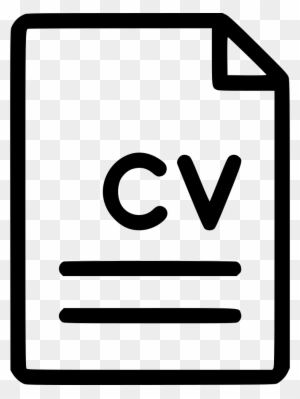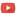KE8QZC | SFW | TSWSyllabus: [pdf] [tex]

Exams
Exam 1: [pdf] [tex]
Exam 2: [pdf] [tex]
Exam 3: [pdf] [tex]

Homework
Homework 1 (due 22 January) (solution: [pdf]) [pdf] [tex]
Homework 2 (due 31 Januarydue 5 February) (solution: [pdf] [tex]): [pdf] [tex]
Homework 3 (due 5 Februarydue 7 February) (solution): [pdf] [tex]
Homework 4 (due 12 February) (solution): [pdf] [tex]
Homework 5 (due 26 February) (solution): [pdf] [tex]
Homework 6 (due 5 March) (solution): [pdf] [tex]
Homework 7 (due 28 March) (solution): [pdf] [tex]
Homework 8 (due 11 April) (solution): [pdf] [tex]
Homework 9 (due 11 April) (solution): [pdf] [tex]
Homework 10 (due 16 April) (solution): [pdf] [tex]
Homework 11 (due 2 May) (solution): [pdf] [tex] (see the 23 April 2019 notes below for definitions of Godel numbers and see 25 April 2019 notes below for definitions of filter)

Quizzes
Quiz 1 (due 24 January) (solution): Show that $P \rightarrow P$ is a tautology using a truth table. Also, show that $Q$ is a propositional consequence of $P \wedge Q$ using a truth table.
Quiz 2 (due 7 February) (solution): Prove $S$ from the premises $P \rightarrow Q$, $Q \rightarrow R$, $\neg R \vee S$, and $P$.
Quiz 3 (due 21 February) (solution): Prove $\forall x(Hx \longleftrightarrow Ix)$ from the premises $\forall x(Hx \rightarrow Ix)$ and $\forall x(Ix \rightarrow Hx)$.
Quiz 4 (due 21 March): Prove the following theorem in naive set theory (see notes from today below): $((x \subseteq y) \wedge (y \subseteq z)) \rightarrow x\subseteq z$.
Quiz 5 (due 15 April): Write the axioms of 3-line geometry and sketch a model of it.
Quiz 6 (due 15 April): Show that the axioms of 3-line geometry are independent by drawing appropriate models.

Notes
notes from 25 April 2019
notes from 23 April 2019
more notes from 11 April 2019
notes from 9 April & 11 April 2019
notes from 19 March 2019
notes from 21 and 26 March 2019
Suppes
Propositional consequences for proofs
Proof example (27 Feb)